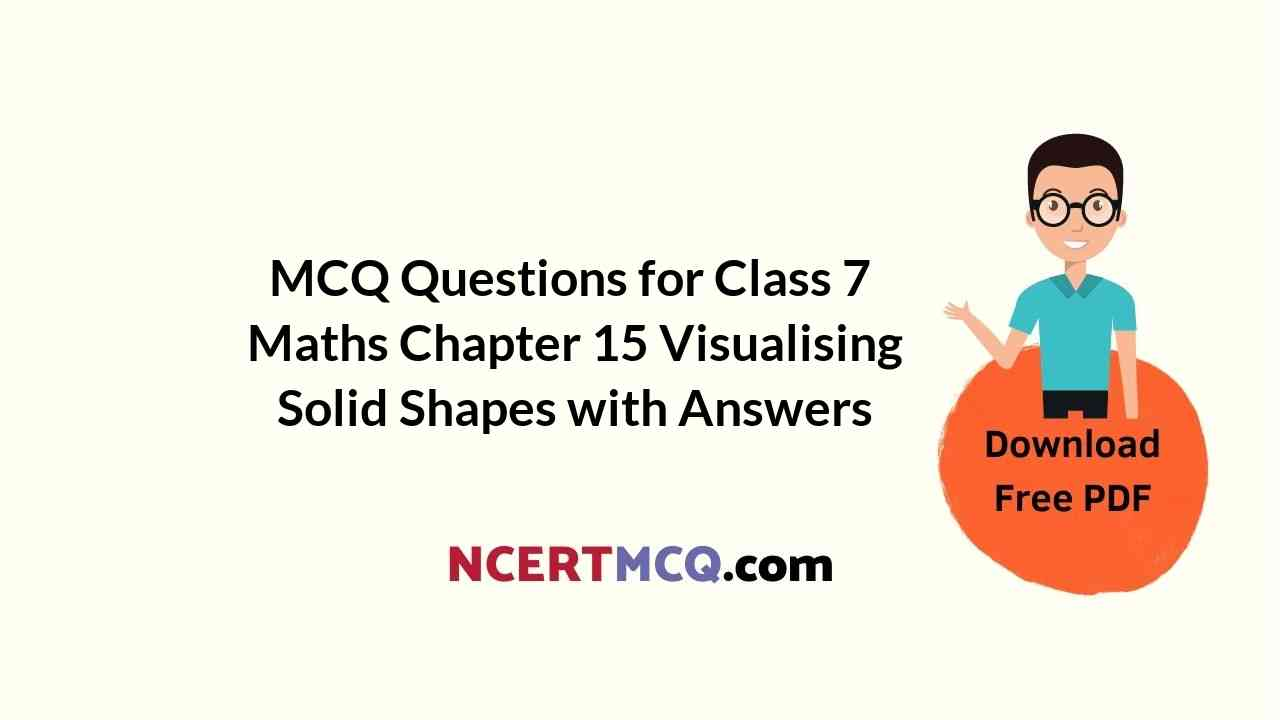Check the below NCERT MCQ Questions for Class 7 Maths Chapter 15 Visualising Solid Shapes with Answers Pdf free download. MCQ Questions for Class 7 Maths with Answers were prepared based on the latest exam pattern. We have provided Visualising Solid Shapes Class 7 Maths MCQs Questions with Answers to help students understand the concept very well. https://ncertmcq.com/mcq-questions-for-class-7-maths-with-answers/

Students can also refer to NCERT Solutions for Class 7 Maths Chapter 15 Visualising Solid Shapes for better exam preparation and score more marks.

## Visualising Solid Shapes Class 7 MCQs Questions with Answers

Visualising Solid Shapes Class 7 MCQ Question 1.
The name of the solid shape is(a) cone
(b) cylinder
(c) sphere
(d) cube

MCQ On Visualising Solid Shapes Class 7 Question 2.
The name of the solid shape is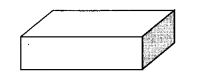(a) cuboid
(b) cube
(c) pyramid
(d) cone

MCQ Questions On Visualising Solid Shapes Class 7 Question 3.
The name of the solid shape is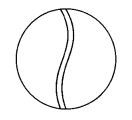(a) cylinder
(b) cone
(c) sphere
(d) cube

Class 7 Maths Chapter 15 MCQ With Answers Question 4.
The name of the solid shape is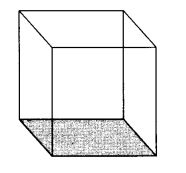(a) cube
(b) cylinder
(c) cone
(d) sphere

Class 7 Maths Chapter 15 Extra Questions Question 5.
The name of the solid shape is(a) cylinder
(b) cone
(c) cuboid
(d) sphere

Visualising Solid Shapes Class 7 Extra Questions Question 6.
The name of the solid shape is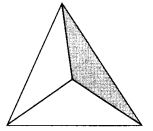(a) cylinder
(b) cone
(c) sphere
(d) pyramid

Class 7 Maths Chapter 15 MCQ Question 7.
The number of vertices of a cube is
(a) 8
(b) 12
(c) 6
(d) 3

MCQ Questions For Class 7 Maths Question 8.
The number of edges of a cube is
(a) 8
(b) 12
(c) 6
(d) 3

MCQ Questions For Class 7 Maths With Answers Pdf Question 9.
The number of faces of a cube is
(a) 8
(b) 12
(c) 6
(d) 3

MCQ Questions Class 7 Maths Question 10.
The number of vertices of the solid shape is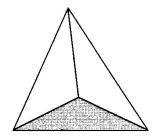(a) 1
(b) 2
(c) 3
(d) 4

Question 11.
The number of faces of the solid shape is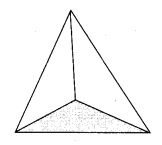(a) 1
(b) 2
(c) 3
(d) 4

Question 12.
The number of edges of the solid shape is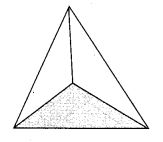(a) 1
(b) 2
(c) 3
(d) 6

Question 13.
The number of vertices of the solid shape is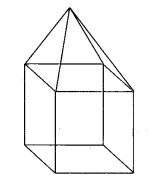(a) 9
(b) 4
(c) 6
(d) 8

Question 14.
The number of faces of the solid shape is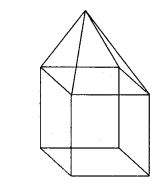(a) 4
(b) 6
(c) 9
(d) 8

Question 15.
The number of edges of the solid shape is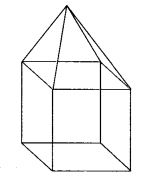(a) 16
(b) 9
(c) 6
(d) 4

Question 16.
Two cubes of edge length 2 cm are placed side by side. The length of the resulting cuboid is
(a) 2 cm
(b) 4 cm
(c) 1 cm
(d) none of these

Question 17.
What cross-section do you get when you give a horizontal cut to a die?
(a) Square
(b) Rectangle
(c) Triangle
(d) Circle

Question 18.
What cross-section do you get when you give a vertical cut to a brick?
(a) Square
(b) Rectangle
(c) Triangle
(d) Circle

Question 19.
What cross-section do you get when you give a horizontal cut to a brick?
(a) Triangle
(b) Circle
(c) Square
(d) Rectangle

Question 20.
What cross-section do you get when you give a vertical cut to a round apple?
(a) Circle
(b) Triangle
(c) Square
(d) Rectangle

Question 21.
What cross-section do you get when you give a horizontal cut to a round apple?
(a) Circle
(b) Square
(c) Rectangle
(d) Triangle

Question 22.
What cross-section do you get when you give a vertical cut to an ice-cream cone?
(a) Triangle
(b) Circle
(c) Rectangle
(d) Square

Question 23.
What cross-section do you get when you give a horizontal cut to an ice-cream cone?
(a) Triangle
(b) Circle
(c) Rectangle
(d) Square

Question 24.
The shadow of the lamp of an a cube when seen under overhead projector is
(a) square
(b) circle
(c) triangle
(d) rectangle